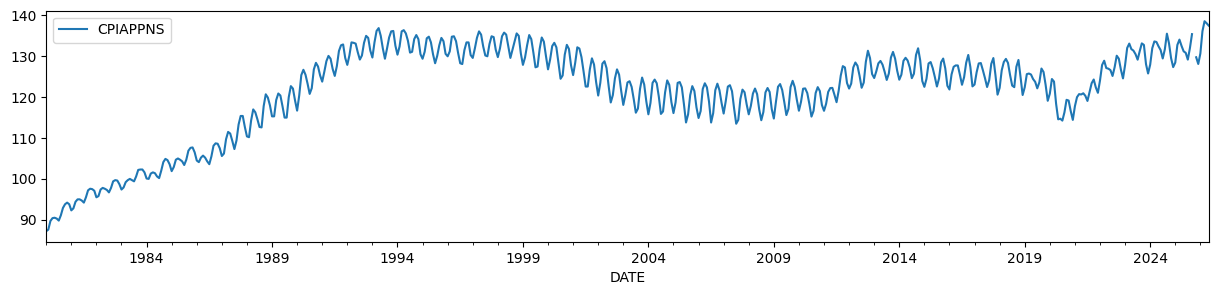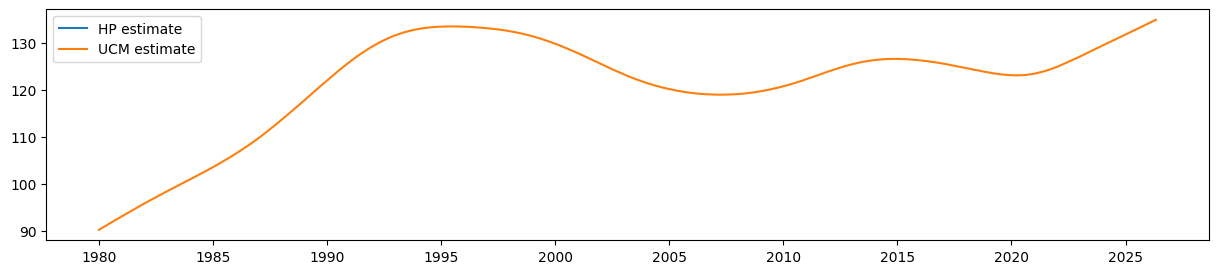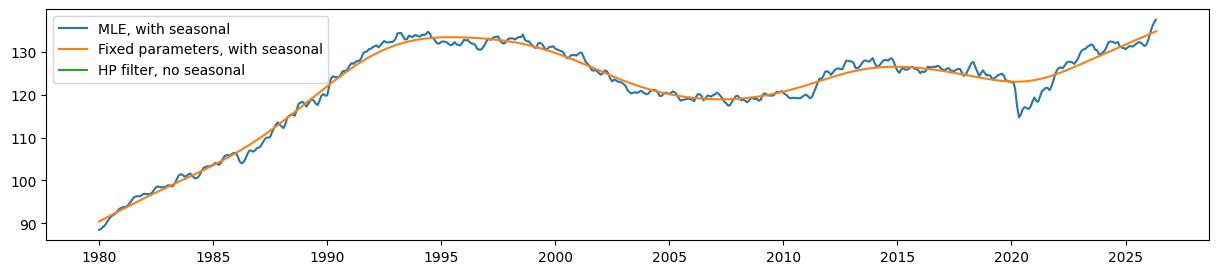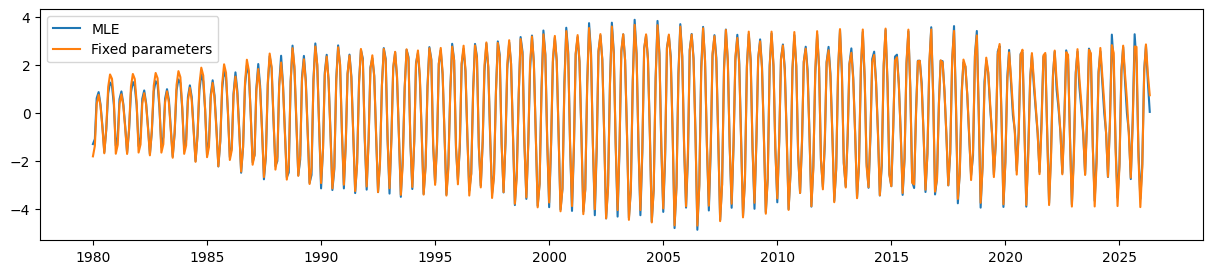# Estimating or specifying parameters in state space models¶

In this notebook we show how to fix specific values of certain parameters in statsmodels’ state space models while estimating others.

In general, state space models allow users to:

1. Estimate all parameters by maximum likelihood

2. Fix some parameters and estimate the rest

3. Fix all parameters (so that no parameters are estimated)

:

%matplotlib inline

import numpy as np
import pandas as pd
import statsmodels.api as sm
import matplotlib.pyplot as plt



To illustrate, we will use the Consumer Price Index for Apparel, which has a time-varying level and a strong seasonal component.

:

endog = DataReader('CPIAPPNS', 'fred', start='1980').asfreq('MS')
endog.plot(figsize=(15, 3));It is well known (e.g. Harvey and Jaeger ) that the HP filter output can be generated by an unobserved components model given certain restrictions on the parameters.

The unobserved components model is:

\begin{split}\begin{aligned} y_t & = \mu_t + \varepsilon_t & \varepsilon_t \sim N(0, \sigma_\varepsilon^2) \\ \mu_t &= \mu_{t-1} + \beta_{t-1} + \eta_t & \eta_t \sim N(0, \sigma_\eta^2) \\ \beta_t &= \beta_{t-1} + \zeta_t & \zeta_t \sim N(0, \sigma_\zeta^2) \\ \end{aligned}\end{split}

For the trend to match the output of the HP filter, the parameters must be set as follows:

\begin{split}\begin{aligned} \frac{\sigma_\varepsilon^2}{\sigma_\zeta^2} & = \lambda \\ \sigma_\eta^2 & = 0 \end{aligned}\end{split}

where $$\lambda$$ is the parameter of the associated HP filter. For the monthly data that we use here, it is usually recommended that $$\lambda = 129600$$.

:

# Run the HP filter with lambda = 129600
hp_cycle, hp_trend = sm.tsa.filters.hpfilter(endog, lamb=129600)

# The unobserved components model above is the local linear trend, or "lltrend", specification
mod = sm.tsa.UnobservedComponents(endog, 'lltrend')
print(mod.param_names)

['sigma2.irregular', 'sigma2.level', 'sigma2.trend']


The parameters of the unobserved components model (UCM) are written as:

• $$\sigma_\varepsilon^2 = \text{sigma2.irregular}$$

• $$\sigma_\eta^2 = \text{sigma2.level}$$

• $$\sigma_\zeta^2 = \text{sigma2.trend}$$

To satisfy the above restrictions, we will set $$(\sigma_\varepsilon^2, \sigma_\eta^2, \sigma_\zeta^2) = (1, 0, 1 / 129600)$$.

Since we are fixing all parameters here, we do not need to use the fit method at all, since that method is used to perform maximum likelihood estimation. Instead, we can directly run the Kalman filter and smoother at our chosen parameters using the smooth method.

:

res = mod.smooth([1., 0, 1. / 129600])
print(res.summary())

                        Unobserved Components Results
==============================================================================
Dep. Variable:               CPIAPPNS   No. Observations:                  520
Model:             local linear trend   Log Likelihood               -2905.346
Date:                Wed, 17 May 2023   AIC                           5816.691
Time:                        23:42:36   BIC                           5829.441
Sample:                    01-01-1980   HQIC                          5821.687
- 04-01-2023
Covariance Type:                  opg
====================================================================================
coef    std err          z      P>|z|      [0.025      0.975]
------------------------------------------------------------------------------------
sigma2.irregular     1.0000      0.009    110.941      0.000       0.982       1.018
sigma2.level              0      0.000          0      1.000      -0.000       0.000
sigma2.trend      7.716e-06   2.05e-07     37.720      0.000    7.32e-06    8.12e-06
===================================================================================
Ljung-Box (L1) (Q):                 242.13   Jarque-Bera (JB):                 1.34
Prob(Q):                              0.00   Prob(JB):                         0.51
Heteroskedasticity (H):               2.38   Skew:                             0.06
Prob(H) (two-sided):                  0.00   Kurtosis:                         2.79
===================================================================================

Warnings:
 Covariance matrix calculated using the outer product of gradients (complex-step).


The estimate that corresponds to the HP filter’s trend estimate is given by the smoothed estimate of the level (which is $$\mu_t$$ in the notation above):

:

ucm_trend = pd.Series(res.level.smoothed, index=endog.index)


It is easy to see that the estimate of the smoothed level from the UCM is equal to the output of the HP filter:

:

fig, ax = plt.subplots(figsize=(15, 3))

ax.plot(hp_trend, label='HP estimate')
ax.plot(ucm_trend, label='UCM estimate')
ax.legend();However, unobserved components models are more flexible than the HP filter. For example, the data shown above is clearly seasonal, but with time-varying seasonal effects (the seasonality is much weaker at the beginning than at the end). One of the benefits of the unobserved components framework is that we can add a stochastic seasonal component. In this case, we will estimate the variance of the seasonal component by maximum likelihood while still including the restriction on the parameters implied above so that the trend corresponds to the HP filter concept.

Adding the stochastic seasonal component adds one new parameter, sigma2.seasonal.

:

# Construct a local linear trend model with a stochastic seasonal component of period 1 year
mod = sm.tsa.UnobservedComponents(endog, 'lltrend', seasonal=12, stochastic_seasonal=True)
print(mod.param_names)

['sigma2.irregular', 'sigma2.level', 'sigma2.trend', 'sigma2.seasonal']


In this case, we will continue to restrict the first three parameters as described above, but we want to estimate the value of sigma2.seasonal by maximum likelihood. Therefore, we will use the fit method along with the fix_params context manager.

The fix_params method takes a dictionary of parameters names and associated values. Within the generated context, those parameters will be used in all cases. In the case of the fit method, only the parameters that were not fixed will be estimated.

:

# Here we restrict the first three parameters to specific values
with mod.fix_params({'sigma2.irregular': 1, 'sigma2.level': 0, 'sigma2.trend': 1. / 129600}):
# Now we fit any remaining parameters, which in this case
# is just sigma2.seasonal
res_restricted = mod.fit()

RUNNING THE L-BFGS-B CODE

* * *

Machine precision = 2.220D-16
N =            1     M =           10

At X0         0 variables are exactly at the bounds

At iterate    0    f=  3.79833D+00    |proj g|=  2.17694D-01

* * *

Tit   = total number of iterations
Tnf   = total number of function evaluations
Tnint = total number of segments explored during Cauchy searches
Skip  = number of BFGS updates skipped
Nact  = number of active bounds at final generalized Cauchy point
Projg = norm of the final projected gradient
F     = final function value

* * *

N    Tit     Tnf  Tnint  Skip  Nact     Projg        F
1      4     11      1     0     0   1.266D-06   3.207D+00
F =   3.2073973914122114


 This problem is unconstrained.


Alternatively, we could have simply used the fit_constrained method, which also accepts a dictionary of constraints:

:

res_restricted = mod.fit_constrained({'sigma2.irregular': 1, 'sigma2.level': 0, 'sigma2.trend': 1. / 129600})

RUNNING THE L-BFGS-B CODE

* * *

Machine precision = 2.220D-16
N =            1     M =           10

At X0         0 variables are exactly at the bounds

At iterate    0    f=  3.79833D+00    |proj g|=  2.17694D-01

 This problem is unconstrained.


* * *

Tit   = total number of iterations
Tnf   = total number of function evaluations
Tnint = total number of segments explored during Cauchy searches
Skip  = number of BFGS updates skipped
Nact  = number of active bounds at final generalized Cauchy point
Projg = norm of the final projected gradient
F     = final function value

* * *

N    Tit     Tnf  Tnint  Skip  Nact     Projg        F
1      4     11      1     0     0   1.266D-06   3.207D+00
F =   3.2073973914122114



The summary output includes all parameters, but indicates that the first three were fixed (and so were not estimated).

:

print(res_restricted.summary())

                            Unobserved Components Results
=====================================================================================
Dep. Variable:                      CPIAPPNS   No. Observations:                  520
Model:                    local linear trend   Log Likelihood               -1667.847
+ stochastic seasonal(12)   AIC                           3337.693
Date:                       Wed, 17 May 2023   BIC                           3341.922
Time:                               23:42:36   HQIC                          3339.352
Sample:                           01-01-1980
- 04-01-2023
Covariance Type:                         opg
============================================================================================
coef    std err          z      P>|z|      [0.025      0.975]
--------------------------------------------------------------------------------------------
sigma2.irregular (fixed)     1.0000        nan        nan        nan         nan         nan
sigma2.level (fixed)              0        nan        nan        nan         nan         nan
sigma2.trend (fixed)      7.716e-06        nan        nan        nan         nan         nan
sigma2.seasonal              0.0957      0.008     12.375      0.000       0.081       0.111
===================================================================================
Ljung-Box (L1) (Q):                 438.73   Jarque-Bera (JB):                45.19
Prob(Q):                              0.00   Prob(JB):                         0.00
Heteroskedasticity (H):               2.60   Skew:                             0.31
Prob(H) (two-sided):                  0.00   Kurtosis:                         4.33
===================================================================================

Warnings:
 Covariance matrix calculated using the outer product of gradients (complex-step).


For comparison, we construct the unrestricted maximum likelihood estimates (MLE). In this case, the estimate of the level will no longer correspond to the HP filter concept.

:

res_unrestricted = mod.fit()

 This problem is unconstrained.

RUNNING THE L-BFGS-B CODE

* * *

Machine precision = 2.220D-16
N =            4     M =           10

At X0         0 variables are exactly at the bounds

At iterate    0    f=  3.63579D+00    |proj g|=  1.06959D-01

At iterate    5    f=  1.98793D+00    |proj g|=  1.22768D+00
ys=-2.835E+00  -gs= 3.191E-01 BFGS update SKIPPED

At iterate   10    f=  1.57447D+00    |proj g|=  6.59470D-01

At iterate   15    f=  1.50678D+00    |proj g|=  1.92466D-01

At iterate   20    f=  1.44226D+00    |proj g|=  2.83519D-01

At iterate   25    f=  1.38790D+00    |proj g|=  2.81915D-02

At iterate   30    f=  1.38738D+00    |proj g|=  5.19229D-05

* * *

Tit   = total number of iterations
Tnf   = total number of function evaluations
Tnint = total number of segments explored during Cauchy searches
Skip  = number of BFGS updates skipped
Nact  = number of active bounds at final generalized Cauchy point
Projg = norm of the final projected gradient
F     = final function value

* * *

N    Tit     Tnf  Tnint  Skip  Nact     Projg        F
4     30     57      1     1     0   5.192D-05   1.387D+00
F =   1.3873774788357895

CONVERGENCE: REL_REDUCTION_OF_F_<=_FACTR*EPSMCH


Finally, we can retrieve the smoothed estimates of the trend and seasonal components.

:

# Construct the smoothed level estimates
unrestricted_trend = pd.Series(res_unrestricted.level.smoothed, index=endog.index)
restricted_trend = pd.Series(res_restricted.level.smoothed, index=endog.index)

# Construct the smoothed estimates of the seasonal pattern
unrestricted_seasonal = pd.Series(res_unrestricted.seasonal.smoothed, index=endog.index)
restricted_seasonal = pd.Series(res_restricted.seasonal.smoothed, index=endog.index)


Comparing the estimated level, it is clear that the seasonal UCM with fixed parameters still produces a trend that corresponds very closely (although no longer exactly) to the HP filter output.

Meanwhile, the estimated level from the model with no parameter restrictions (the MLE model) is much less smooth than these.

:

fig, ax = plt.subplots(figsize=(15, 3))

ax.plot(unrestricted_trend, label='MLE, with seasonal')
ax.plot(restricted_trend, label='Fixed parameters, with seasonal')
ax.plot(hp_trend, label='HP filter, no seasonal')
ax.legend();Finally, the UCM with the parameter restrictions is still able to pick up the time-varying seasonal component quite well.

:

fig, ax = plt.subplots(figsize=(15, 3))

ax.plot(unrestricted_seasonal, label='MLE')
ax.plot(restricted_seasonal, label='Fixed parameters')
ax.legend();Last update: May 17, 2023